4.2.2 Solved Forms of Dominance Constraints

If a dominance constraint is satisfiable, it has infinitely many models; in particular, if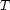is a solution of, then any tree that containsis also a solution. For example, here are a few models of: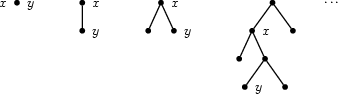The problem is similar when solving equations on first-order terms. The equation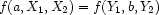has infinitely many models, namely all first-order terms of the form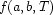whereis a first-order term. Instead of attempting to enumerate all models, a solver returns a most general unifier: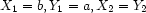A unifier is also known as a solved form: it represents a non-empty family of solutions.

For a dominance constraint, we are going to proceed similarly and search for solved forms rather than for solution trees. The idea is that we simply want to arrange the nodes interpreting the variables ofinto a tree shape.

For our purpose, a solved form will make explicit the relationship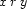that holds between every two variablesandof the description: i.e. in a solved form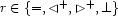. In [DN00], we show that it is possible to use less explicit solved forms while preserving completeness: the relationship betweenandneed not be fully decided, but merely one ofor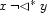. For simplicity of presentation, we will only consider fully explicit solved forms, but, in practice, less explicit solved forms are to be preferred since they require less search. In [DN00], we show that the less explicit solved forms may avoid an exponential number of choice points.

Denys Duchier
Version 1.2.0 (20010221)